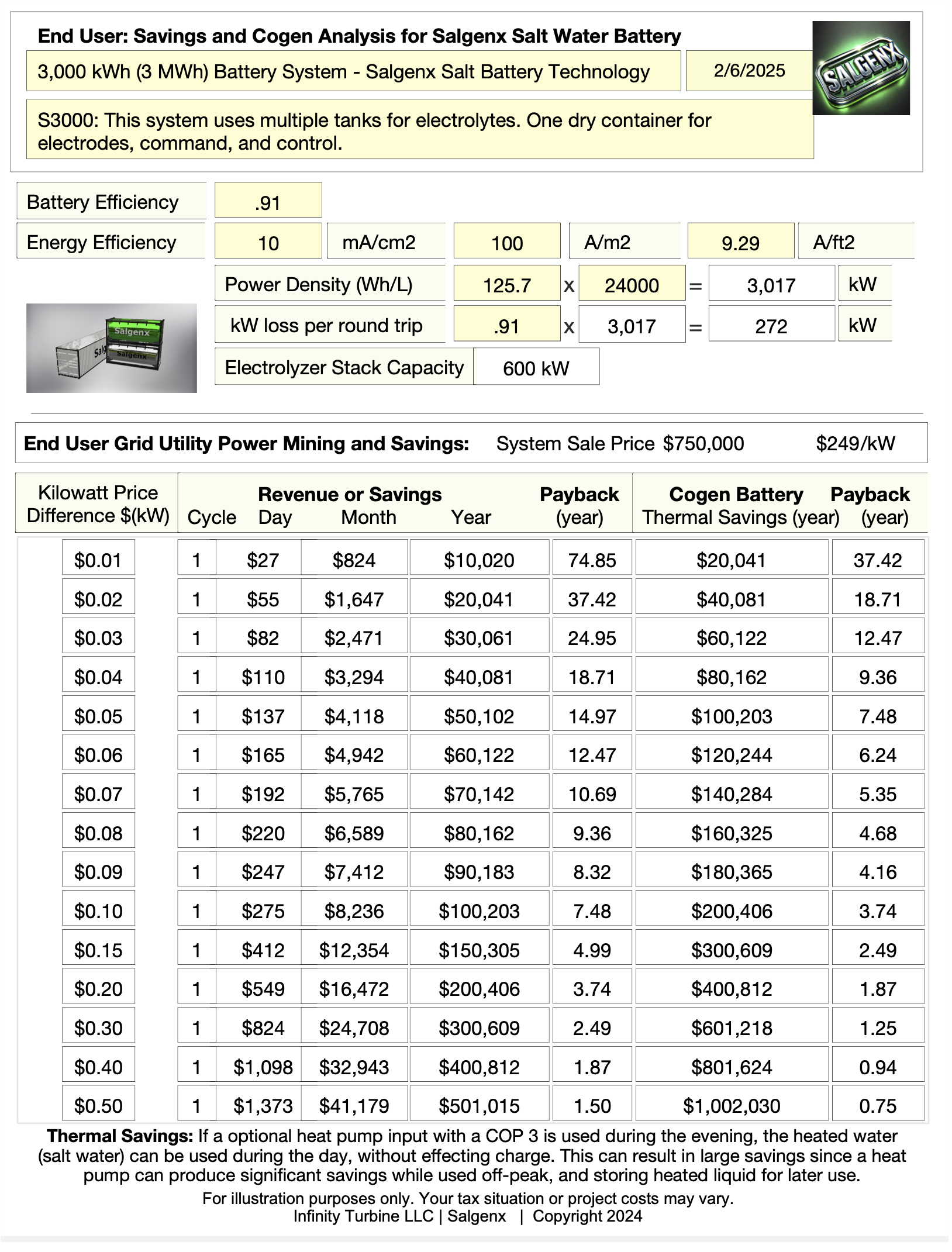# Salt Water Flow Battery CogenerationThermal Applications Salgenx S3000 grid power mining and thermal savings chart.With a COP of 5, what are the energy savings of a heat pump when grid power is at \$.15 per kwh ? A heat pump with a coefficient of performance (COP) of 5 means that for every unit of electrical energy input, the heat pump can produce 5 units of heat energy. This means that a heat pump with a COP of 5 is five times more efficient at producing heat energy than an electric resistance heater, which has a COP of 1.To calculate the energy savings of a heat pump, you need to know the cost of the electricity and the amount of electricity used.If the cost of grid power is \$0.15 per kWh and the heat pump is used to generate 1kWh of heat energy, the savings would be:1kWh x \$0.15/kWh = \$0.15 (cost of electricity to generate 1kWh of heat energy with an electric resistance heater)1kWh / 5 (COP) = 0.2kWh (amount of electricity used to generate 1kWh of heat energy with the heat pump)0.2kWh x \$0.15/kWh = \$0.03 (cost of electricity to generate 1kWh of heat energy with the heat pump)Therefore, the energy savings with a heat pump would be:\$0.15 - \$0.03 = \$0.12It's worth noting that this is a simplified calculation and that the actual energy savings will depend on a number of factors, such as the specific heat pump, the temperature of the heat source, and the specific application. Additionally, the savings will depend on the price of electricity, which can vary depending on the location and the time of day.Overall, heat pumps are highly efficient devices that can provide significant energy savings, especially when the grid power is expensive.
 CONTACT TEL: 608-238-6001 Email: greg@salgenx.com | RSS | AMP | PDF | IG